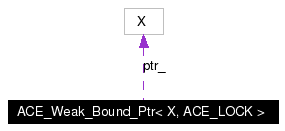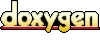# ACE_Weak_Bound_Ptr< X, ACE_LOCK > Class Template Reference

This class implements support for a weak pointer that complements ACE_Strong_Bound_Ptr. More...

`#include <Bound_Ptr.h>`

Collaboration diagram for ACE_Weak_Bound_Ptr< X, ACE_LOCK >:[legend]
List of all members.

## Public Member Functions

ACE_Weak_Bound_Ptr (X *p=0)
ACE_Weak_Bound_Ptr (const ACE_Weak_Bound_Ptr< X, ACE_LOCK > &r)
Copy constructor binds and to the same object.

ACE_Weak_Bound_Ptr (const ACE_Strong_Bound_Ptr< X, ACE_LOCK > &r)
Constructor binds and to the same object.

~ACE_Weak_Bound_Ptr (void)
Destructor.

void operator= (const ACE_Weak_Bound_Ptr< X, ACE_LOCK > &r)
Assignment operator that binds and to the same object.

void operator= (const ACE_Strong_Bound_Ptr< X, ACE_LOCK > &r)
Assignment operator that binds and to the same object.

bool operator== (const ACE_Weak_Bound_Ptr< X, ACE_LOCK > &r) const
bool operator== (const ACE_Strong_Bound_Ptr< X, ACE_LOCK > &r) const
bool operator== (X *p) const
bool operator!= (const ACE_Weak_Bound_Ptr< X, ACE_LOCK > &r) const
Inequality operator, which is the opposite of equality.

bool operator!= (const ACE_Strong_Bound_Ptr< X, ACE_LOCK > &r) const
Inequality operator, which is the opposite of equality.

bool operator!= (X *p) const
Inequality operator, which is the opposite of equality.

ACE_Strong_Bound_Ptr< X, ACE_LOCK > operator-> (void) const
Redirection operator.

ACE_Strong_Bound_Ptr< X, ACE_LOCK > strong (void) const
X * unsafe_get (void) const
void reset (X *p=0)
Increment the reference count on the underlying object.

int remove_ref (void)
int null (void) const
Allows us to check for NULL on all ACE_Weak_Bound_Ptr objects.

## Public Attributes

ACE_ALLOC_HOOK_DECLARE
Declare the dynamic allocation hooks.

## Private Types

typedef ACE_Bound_Ptr_Counter<
ACE_LOCK >
COUNTER
The ACE_Bound_Ptr_Counter type.

## Private Attributes

COUNTERcounter_
The reference counter.

X * ptr_
The underlying object.

## Friends

class ACE_Strong_Bound_Ptr< X, ACE_LOCK >

## Detailed Description

### template<class X, class ACE_LOCK> class ACE_Weak_Bound_Ptr< X, ACE_LOCK >

This class implements support for a weak pointer that complements ACE_Strong_Bound_Ptr.

Unlike ACE_Strong_Bound_Ptr, assigning or copying instances of an ACE_Weak_Bound_Ptr will not automatically increment the reference count of the underlying object. What ACE_Weak_Bound_Ptr does is preserve the knowledge that the object is in fact reference counted, and thus provides an alternative to raw pointers where non-ownership associations must be maintained. When the last instance of an ACE_Strong_Bound_Ptr that references a particular object is destroyed or overwritten, the corresponding ACE_Weak_Bound_Ptr instances are set to NULL.

Definition at line 224 of file Bound_Ptr.h.

## Member Typedef Documentation

 template typedef ACE_Bound_Ptr_Counter ACE_Weak_Bound_Ptr< X, ACE_LOCK >::COUNTER` [private]`
 The ACE_Bound_Ptr_Counter type. Definition at line 329 of file Bound_Ptr.h.

## Constructor & Destructor Documentation

 template ACE_Weak_Bound_Ptr< X, ACE_LOCK >::ACE_Weak_Bound_Ptr ( X * p = 0 ) ` [inline, explicit]`
 Constructor that initializes an ACE_Weak_Bound_Ptr to point to the object

immediately. Definition at line 314 of file Bound_Ptr.inl. ```00315 : counter_ (COUNTER::create_weak ()), 00316 ptr_ (p) 00317 { 00318 } ```

 template ACE_Weak_Bound_Ptr< X, ACE_LOCK >::ACE_Weak_Bound_Ptr ( const ACE_Weak_Bound_Ptr< X, ACE_LOCK > & r ) ` [inline]`
 Copy constructor binds and to the same object. Definition at line 321 of file Bound_Ptr.inl. ```00322 : counter_ (r.counter_), 00323 ptr_ (r.ptr_) 00324 { 00325 COUNTER::attach_weak (this->counter_); 00326 } ```

 template ACE_Weak_Bound_Ptr< X, ACE_LOCK >::ACE_Weak_Bound_Ptr ( const ACE_Strong_Bound_Ptr< X, ACE_LOCK > & r ) ` [inline]`
 Constructor binds and to the same object. Definition at line 329 of file Bound_Ptr.inl. ```00330 : counter_ (r.counter_), 00331 ptr_ (r.ptr_) 00332 { 00333 COUNTER::attach_weak (this->counter_); 00334 } ```

 template ACE_Weak_Bound_Ptr< X, ACE_LOCK >::~ACE_Weak_Bound_Ptr ( void ) ` [inline]`
 Destructor. Definition at line 337 of file Bound_Ptr.inl. ```00338 { 00339 COUNTER::detach_weak (this->counter_); 00340 } ```

## Member Function Documentation

 template int ACE_Weak_Bound_Ptr< X, ACE_LOCK >::add_ref ( void ) ` [inline]`
 Increment the reference count on the underlying object. Returns the new reference count on the object. This function may be used to integrate the bound pointers into an external reference counting mechanism such as those used by COM or CORBA servants. Definition at line 471 of file Bound_Ptr.inl. ```00472 { 00473 return COUNTER::attach_strong (counter_); 00474 } ```

 template int ACE_Weak_Bound_Ptr< X, ACE_LOCK >::null ( void ) const` [inline]`
 Allows us to check for NULL on all ACE_Weak_Bound_Ptr objects. Definition at line 451 of file Bound_Ptr.inl. ```00452 { 00453 // A weak pointer must behave as though it is automatically set to null 00454 // if the underlying object has been deleted. 00455 if (COUNTER::object_was_deleted (this->counter_)) 00456 return 1; 00457 00458 return this->ptr_ == 0; 00459 } ```

 template bool ACE_Weak_Bound_Ptr< X, ACE_LOCK >::operator!= ( X * p ) const` [inline]`
 Inequality operator, which is the opposite of equality. Definition at line 420 of file Bound_Ptr.inl. ```00421 { 00422 // A weak pointer must behave as though it is automatically set to null 00423 // if the underlying object has been deleted. 00424 if (COUNTER::object_was_deleted (this->counter_)) 00425 return p != 0; 00426 00427 return this->ptr_ != p; 00428 } ```

 template bool ACE_Weak_Bound_Ptr< X, ACE_LOCK >::operator!= ( const ACE_Strong_Bound_Ptr< X, ACE_LOCK > & r ) const` [inline]`
 Inequality operator, which is the opposite of equality. Definition at line 409 of file Bound_Ptr.inl. References ACE_Strong_Bound_Ptr< X, ACE_LOCK >::ptr_. ```00410 { 00411 // A weak pointer must behave as though it is automatically set to null 00412 // if the underlying object has been deleted. 00413 if (COUNTER::object_was_deleted (this->counter_)) 00414 return r.ptr_ != 0; 00415 00416 return this->ptr_ != r.ptr_; 00417 } ```

 template bool ACE_Weak_Bound_Ptr< X, ACE_LOCK >::operator!= ( const ACE_Weak_Bound_Ptr< X, ACE_LOCK > & r ) const` [inline]`
 Inequality operator, which is the opposite of equality. Definition at line 398 of file Bound_Ptr.inl. References ACE_Weak_Bound_Ptr< X, ACE_LOCK >::ptr_. ```00399 { 00400 // A weak pointer must behave as though it is automatically set to null 00401 // if the underlying object has been deleted. 00402 if (COUNTER::object_was_deleted (this->counter_)) 00403 return r.ptr_ != 0; 00404 00405 return this->ptr_ != r.ptr_; 00406 } ```

 template ACE_Strong_Bound_Ptr< X, ACE_LOCK > ACE_Weak_Bound_Ptr< X, ACE_LOCK >::operator-> ( void ) const` [inline]`
 Redirection operator. It returns a temporary strong pointer and makes use of the chaining properties of operator-> to ensure that the underlying object does not disappear while you are using it. If you are certain of the lifetimes of the object, and do not want to incur the locking overhead, then use the unsafe_get method instead. Definition at line 431 of file Bound_Ptr.inl. ```00432 { 00433 return ACE_Strong_Bound_Ptr (*this); 00434 } ```

 template void ACE_Weak_Bound_Ptr< X, ACE_LOCK >::operator= ( const ACE_Strong_Bound_Ptr< X, ACE_LOCK > & r ) ` [inline]`
 Assignment operator that binds and to the same object. Definition at line 354 of file Bound_Ptr.inl. ```00355 { 00356 // This will work if &rhs == this, by first increasing the ref count 00357 COUNTER *new_counter = rhs.counter_; 00358 COUNTER::attach_weak (new_counter); 00359 COUNTER::detach_weak (this->counter_); 00360 this->counter_ = new_counter; 00361 this->ptr_ = rhs.ptr_; 00362 } ```

 template void ACE_Weak_Bound_Ptr< X, ACE_LOCK >::operator= ( const ACE_Weak_Bound_Ptr< X, ACE_LOCK > & r ) ` [inline]`
 Assignment operator that binds and to the same object. Definition at line 343 of file Bound_Ptr.inl. ```00344 { 00345 // This will work if &rhs == this, by first increasing the ref count 00346 COUNTER *new_counter = rhs.counter_; 00347 COUNTER::attach_weak (new_counter); 00348 COUNTER::detach_weak (this->counter_); 00349 this->counter_ = new_counter; 00350 this->ptr_ = rhs.ptr_; 00351 } ```

 template bool ACE_Weak_Bound_Ptr< X, ACE_LOCK >::operator== ( X * p ) const` [inline]`
 Equality operator that returns `true` if the ACE_Weak_Bound_Ptr and the raw pointer point to the same underlying object. Definition at line 387 of file Bound_Ptr.inl. ```00388 { 00389 // A weak pointer must behave as though it is automatically set to null 00390 // if the underlying object has been deleted. 00391 if (COUNTER::object_was_deleted (this->counter_)) 00392 return p == 0; 00393 00394 return this->ptr_ == p; 00395 } ```

 template bool ACE_Weak_Bound_Ptr< X, ACE_LOCK >::operator== ( const ACE_Strong_Bound_Ptr< X, ACE_LOCK > & r ) const` [inline]`
 Note:It also returns `true` if both objects have just been instantiated and not used yet. Definition at line 376 of file Bound_Ptr.inl. References ACE_Strong_Bound_Ptr< X, ACE_LOCK >::ptr_. ```00377 { 00378 // A weak pointer must behave as though it is automatically set to null 00379 // if the underlying object has been deleted. 00380 if (COUNTER::object_was_deleted (this->counter_)) 00381 return r.ptr_ == 0; 00382 00383 return this->ptr_ == r.ptr_; 00384 } ```

 template bool ACE_Weak_Bound_Ptr< X, ACE_LOCK >::operator== ( const ACE_Weak_Bound_Ptr< X, ACE_LOCK > & r ) const` [inline]`
 Note:It also returns `true` if both objects have just been instantiated and not used yet. Definition at line 365 of file Bound_Ptr.inl. References ACE_Weak_Bound_Ptr< X, ACE_LOCK >::ptr_. ```00366 { 00367 // A weak pointer must behave as though it is automatically set to null 00368 // if the underlying object has been deleted. 00369 if (COUNTER::object_was_deleted (this->counter_)) 00370 return r.ptr_ == 0; 00371 00372 return this->ptr_ == r.ptr_; 00373 } ```

 template int ACE_Weak_Bound_Ptr< X, ACE_LOCK >::remove_ref ( void ) ` [inline]`
 Returns the new reference count on the object. This function may be used to integrate the bound pointers into an external reference counting mechanism such as those used by COM or CORBA servants. Definition at line 477 of file Bound_Ptr.inl. ```00478 { 00479 int new_obj_ref_count = COUNTER::detach_strong (counter_); 00480 if (new_obj_ref_count == 0) 00481 { 00482 delete this->ptr_; 00483 this->ptr_ = 0; 00484 } 00485 return new_obj_ref_count; 00486 } ```

 template void ACE_Weak_Bound_Ptr< X, ACE_LOCK >::reset ( X * p = 0 ) ` [inline]`
 Resets the ACE_Weak_Bound_Ptr to refer to a different underlying object. Definition at line 462 of file Bound_Ptr.inl. ```00463 { 00464 COUNTER *old_counter = this->counter_; 00465 this->counter_ = COUNTER::create_weak (); 00466 this->ptr_ = p; 00467 COUNTER::detach_weak (old_counter); 00468 } ```

 template ACE_Strong_Bound_Ptr< X, ACE_LOCK > ACE_Weak_Bound_Ptr< X, ACE_LOCK >::strong ( void ) const` [inline]`
 Obtain a strong pointer corresponding to this weak pointer. This function is useful to create a temporary strong pointer for conversion to a reference. Definition at line 437 of file Bound_Ptr.inl. ```00438 { 00439 return ACE_Strong_Bound_Ptr (*this); 00440 } ```

 template X * ACE_Weak_Bound_Ptr< X, ACE_LOCK >::unsafe_get ( void ) const` [inline]`
 Get the pointer value. Warning: this does not affect the reference count of the underlying object, so it may disappear on you while you are using it if you are not careful. Definition at line 443 of file Bound_Ptr.inl. ```00444 { 00445 // We do not check if the object has been deleted, since this operation 00446 // is defined to be unsafe! 00447 return this->ptr_; 00448 } ```

## Friends And Related Function Documentation

 template friend class ACE_Strong_Bound_Ptr< X, ACE_LOCK >` [friend]`
 Definition at line 326 of file Bound_Ptr.h.

## Member Data Documentation

 template ACE_Weak_Bound_Ptr< X, ACE_LOCK >::ACE_ALLOC_HOOK_DECLARE
 Declare the dynamic allocation hooks. Definition at line 323 of file Bound_Ptr.h.

 template COUNTER* ACE_Weak_Bound_Ptr< X, ACE_LOCK >::counter_` [private]`
 The reference counter. Definition at line 332 of file Bound_Ptr.h.

 template X* ACE_Weak_Bound_Ptr< X, ACE_LOCK >::ptr_` [private]`
 The underlying object. Definition at line 335 of file Bound_Ptr.h.

The documentation for this class was generated from the following files:
Generated on Thu Nov 9 11:32:25 2006 for ACE by1.3.6